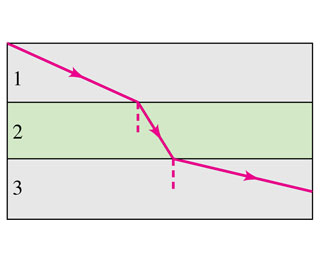# Problem: Parallel light rays cross interfaces from medium 1 into medium 2 and then into medium 3 as shown in(Figure 1).Part A. What can we say about the relative sizes of the indices of refraction of these media?a. n3 &gt; n2 &gt; n1.b. n2 &gt; n1 &gt; n3.c. n2 &gt; n3 &gt; n1.d. n1 &gt; n2 &gt; n3.e. n1 &gt; n3 &gt; n2.f. None of the above.

###### FREE Expert Solution

We'll use Snel's law in this problem.

Snell's law is used to describe the relationship between the incident angle, refracted angle, and refractive indices of two media.

Snell's law:

$\overline{){{\mathbf{\eta }}}_{{\mathbf{1}}}{\mathbf{s}}{\mathbf{i}}{\mathbf{n}}{{\mathbf{\theta }}}_{{\mathbf{1}}}{\mathbf{=}}{{\mathbf{\eta }}}_{{\mathbf{2}}}{\mathbf{s}}{\mathbf{i}}{\mathbf{n}}{{\mathbf{\theta }}}_{{\mathbf{2}}}}$, where n1 and n2 are refractive indices of medium 1 and medium 2. θ1 and θ2 are the incident and refractive angles, respectively.

Rearranging Snell's law gives:

$\frac{{\mathbf{\eta }}_{\mathbf{1}}}{{\mathbf{\eta }}_{\mathbf{2}}}\mathbf{=}\frac{\mathbf{s}\mathbf{i}\mathbf{n}{\mathbf{\theta }}_{\mathbf{2}}}{\mathbf{s}\mathbf{i}\mathbf{n}{\mathbf{\theta }}_{\mathbf{1}}}$

97% (459 ratings)###### Problem Details

Parallel light rays cross interfaces from medium 1 into medium 2 and then into medium 3 as shown in(Figure 1).Part A. What can we say about the relative sizes of the indices of refraction of these media?
a. n3 > n2 > n1.
b. n2 > n1 > n3.
c. n2 > n3 > n1.
d. n1 > n2 > n3.
e. n1 > n3 > n2.
f. None of the above.